0%

## 地图分类

• 尺度地图（Matric Map）：在尺度地图中，每一个地点可以用一个坐标来表示，例如经纬度地图。栅格地图也是尺度地图的一种，在栅格地图中，环境被分割成一个个栅格；尺度地图中的距离和真实环境的距离是成一定比例的
• 拓扑地图（Topological Map）：拓扑地图由边和点组成，每个地点用一个点表示，地点之间的距离用边表示；相对于尺度地图，拓扑地图搜索速度更快，因为在尺度地图中我们必须通过不断搜索周围栅格来进行对目标搜索，哪怕当大部分栅格都是空的情况也需要这么操作，而在拓扑地图中，我们只需要搜索周围的点，来判断两个点之间是否有连通通路即可
• 语义地图（Semantic Map）：前两种地图对地点的表示方法都是点（栅格或者节点），而语义地图在这个点的基础添加标签

## 覆盖栅格建图算法

### 特点

• 将环境分解成一个个栅格
• 每个栅格有两种状态：占用（Occupied）、空闲(Free)，某些情况下我们还会添加未知（Unknown） 也作为栅格状态的一种，表明不知道该栅格的占用情况
• 整个地图是一个非参模型，只用一系列孤立的栅格组成，没有通过某些参数来建模
• 天然区分可通行区域（栅格状态即可表示），适合进行轨迹规划
• 由于环境中每个点都需要用栅格表示，因此内存需求随地图增大急剧增加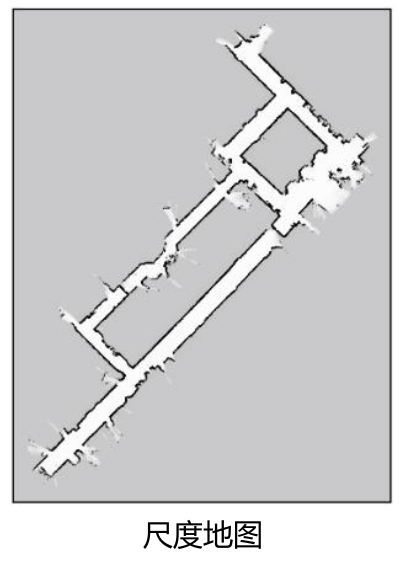### 待解决问题-数学描述

• $p(m_i) = 1$ 表示占用
• $p(m_i) = 0$ 表示空闲

### 地图栅格估计过程

• 每帧激光数据独立，$z_i$ 之间相互不关联
• 每帧激光数据只与该一时刻位姿相关，即 $z_i$ 只与 $x_i$ 只在 $i$ 相等时相关
• 每个栅格 $m_i$ 与每一对 $x_i$ 和 $z_i$ 同时相关，与单一项不相关（例如只有位姿没有观测）

• $l(m_i)$: 栅格 $m_i$ 的先验值，我们对所有栅格可以设定同样一个初始值
• $l(mi|z{1:t-1},x_{1:t-1})$:
• 在 $t - 1$ 时刻的数据时 $m_i$ 的状态
• $l(m_i|z_t,x_t)$: 传感器的逆观测模型 （inverse measurement model），因为正常的观测模型为给定 $t$ 时刻的地图 $m_i$ 和位姿 $x_t$ 对 $z_t$ 的结果，而这里是反过来的

• 不经过该栅格，$l(m_i|z_t,x_t) = 0$ 不产生影响，在这一时刻不会处理这个栅格
• 经过栅格没受到阻拦，$l(m_i|z_t,x_t) = -a$，由于穿过去了，表面该位置有障碍物的可能性比较低，因此我们用负值表示，$a$ 的大小取决于我们对其的相信程度
• 经过栅格被阻拦了，$l(m_i|z_t,x_t) = +b$，和上述类似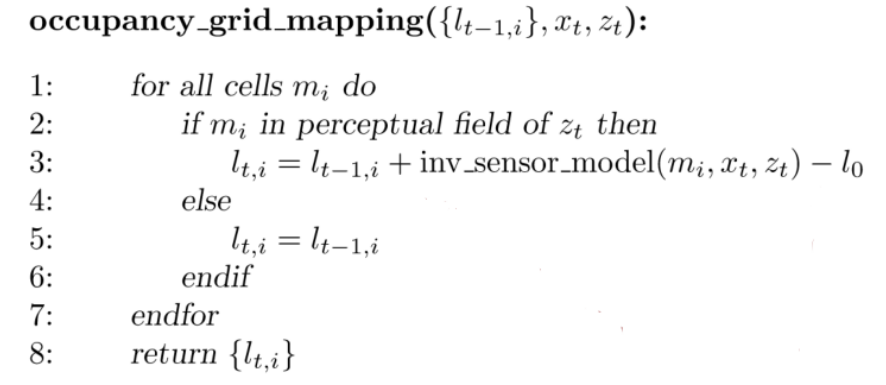## 计数建图算法

### 基本概念

• 对每一个栅格 $m_i$ 统计两个量: misses(i)hits(i)，其中：
• misses(i) 表示 i 栅格被通过（即被标为 free）的次数
• hits(i) 表示 i 被激光束击中（即标为 occupied）的次数
• hits(i) / (misses(i) + hits(i)) 表示 i 状态的极大似然估计（下面进行证明），当其超过一定阈值时则认为该栅格为 occupied,否则为 free

### 激光雷达观测模型

• $x_t$：$t$ 时刻的机器人位姿
• $z_t$：$t$ 时刻的激光数据
• 可以理解为一个数组，每一束激光束的数组 $z{t, i}$ 表示第 $i$ 个激光束最终停在该方向上第 $z{t, i}$ 个栅格上
• 第 n 个激光束沿着射线方向一共穿过 $z_{t,n} - 1$ 个栅格，击中 $1$ 个栅格
• $c_{t,n}$:
• 表示 $t$ 时刻的第 $n$ 个激光束是否为最大值，即达到激光测距极限，此时该距离为无效值，不能表示 该方向上第 $z_{t, n}$ 个栅格有障碍物
• $c{t,n} = 1$ 表示达到最大值，$c{t,n} = 0$ 表示正常值
• $f(xt, n, z{t,n})$：表示 $t$ 时刻第 $n$ 个激光束击中的栅格的下标，因此 $m{f(x_t, n, z{t,n})}$ 为该栅格的占用概率，通过上述的分析可以得出：

• 返回无效（最大）距离时：在给定的位姿 $xt$ 和地图 $m$ 的条件下，在该方向击中第 $z{t,n}$ 个栅格的概率为：该方向前 $z_{t,n} - 1$ 个栅格都为 free 的概率，这个时候我们不用考虑最后一个栅格是否有障碍物，因为无论有没有激光束最远也只能到那个栅格
• 返回有效值时，除了要满足前 $z{t,n} - 1$ 个栅格都为 free 的条件外，还必须要乘上第 $z{t,n}$ 为 occupied 的概率，因为只有当它是 occupied 才会击中该栅格

### 地图估计的数学描述

• $\sum_t$ 表示遍历所有激光帧（时刻）
• $\sum_j$ 表示遍历所有栅格位置
• $\sum_n$ 表示遍历一个激光帧的所有激光束

• 这表示了所有激光帧中所有激光束正常击中（不是在极限距离）$m_j$ 栅格的次数 $hits(j)$
• 表示栅格 $m_j$ 被所有激光帧中所有激光束穿过的次数 $miss(j)$

$F(m_j)$是个简单函数，可以直接对其求导且令导数为 0：

## TSDF（Truncated signed distance function） 建图算法

### 基本思想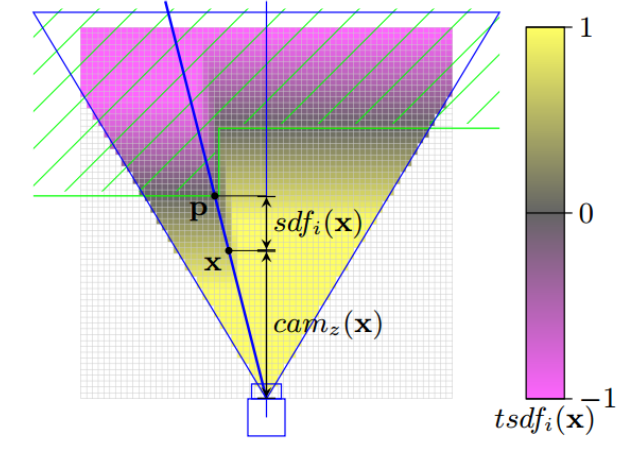• 考虑传感器测量的不确定性，利用多次测量数据来实现更为精确的表面重构，从而使得地图更精确、障碍物（墙壁）更细、更薄
• 为障碍物表面建立 Signed Distance Function （有符号距离函数）
• 考虑到距离表面较远的点不会影响到表面重构，对其忽略不计

### TSDF

• $laser_i(x)$ 表示激光测量距离
• $dist_i(x)$ 表示栅格离传感器原点的距离

### TSDF 实例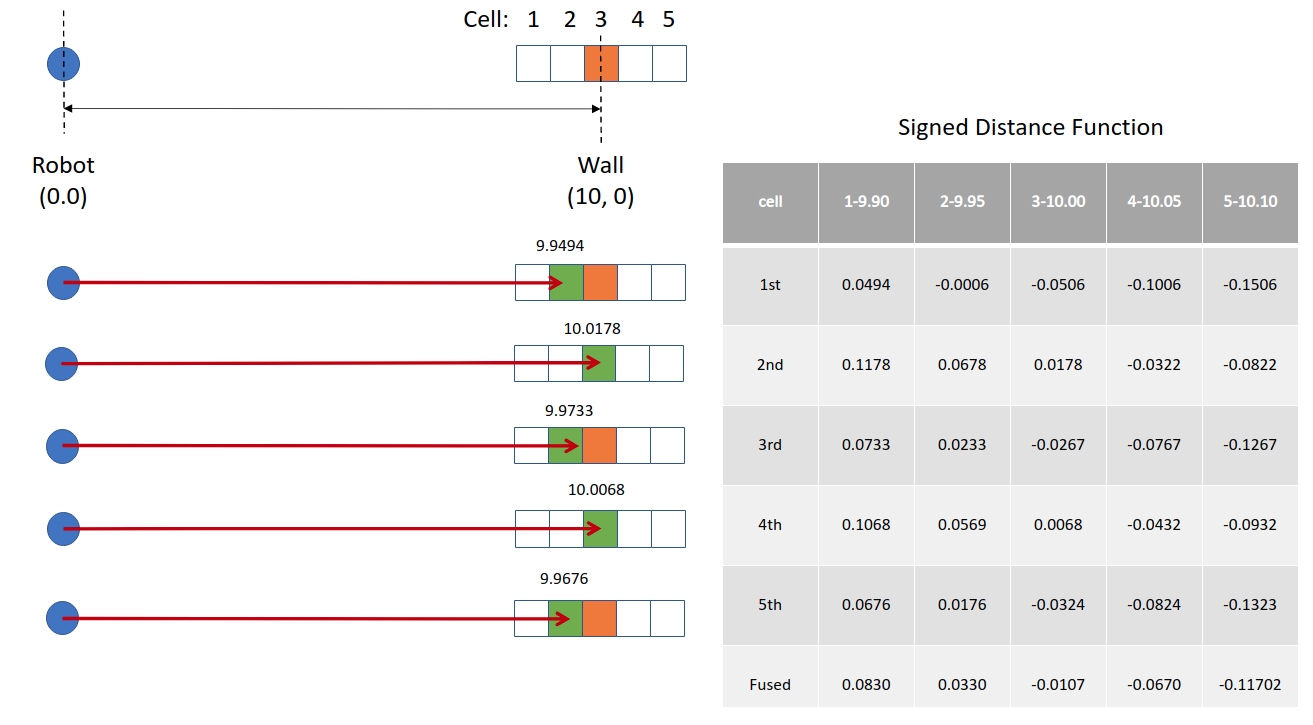### TSDF 算法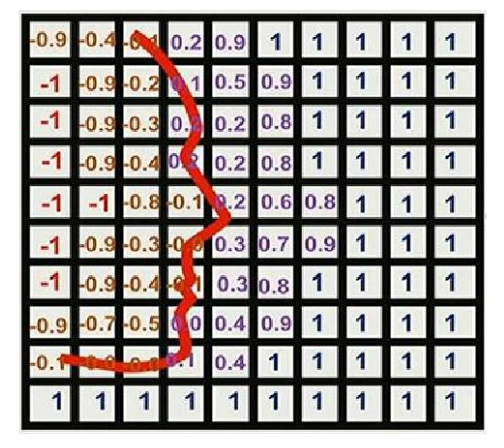• 寻找 TSDF 场中符号进行变化的栅格，进曲面所在位置
• 在该两个栅格之间进行插值，插值得到 0 的坐标就是曲面精确位置
• 融合多帧观测，也相当于对多帧观测数据进行加权最小二乘求解
• 由于我们是通过插值得到障碍区曲面，所以构建的地图最多只有一个栅格厚度